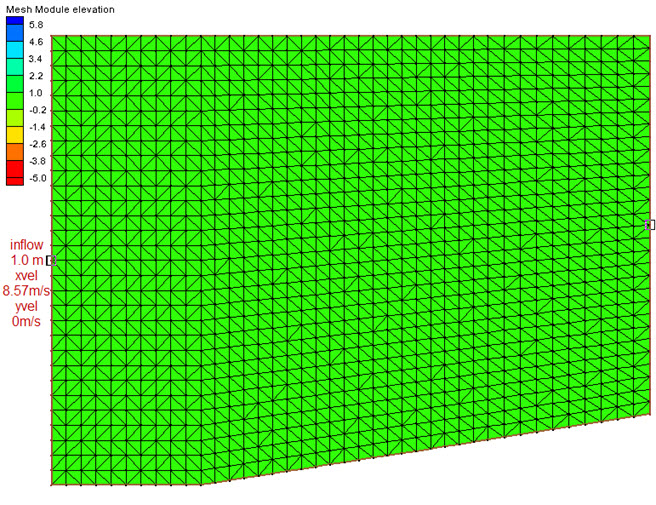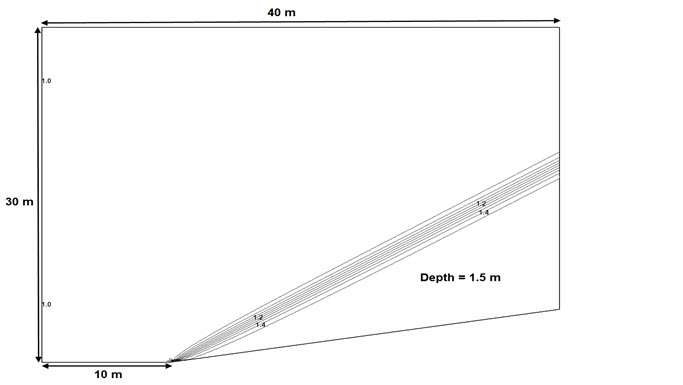## Convergent Wall

Reference

• Choi et. al; "A Spectral Finite Volume Model for Shallow Water Equations". Journal of the American Meteorological Society , July 2004, 1777-1791.

Domain

• rectangular flume
• 40 m length
• 30 m width
• 8.6 deg angle with the horizontal at x=10 m on the bottom edgeInitial Conditions

• no flow
• 1.0 m depth

Boundary Conditions

• 8.57 m/s x-velocity, 0 m/s y-velocity at inflow (left)
• Froude number = 2.74 at inflow (left)
• supercritical outflow (right)

Run Files

• 2D-convergent_wall.3dm
• 2D-convergent_wall.bc
• 2D-convergent_wall.hot

Results

• Model run to steady state
• Hydraulic jump forms at bottom edge transition point along a 30 deg angle
• Analytic Results give:
• downstream depth = 1.5 m
• downstream Froude number = 2.075
• jump angle is 30 deg with x-direction